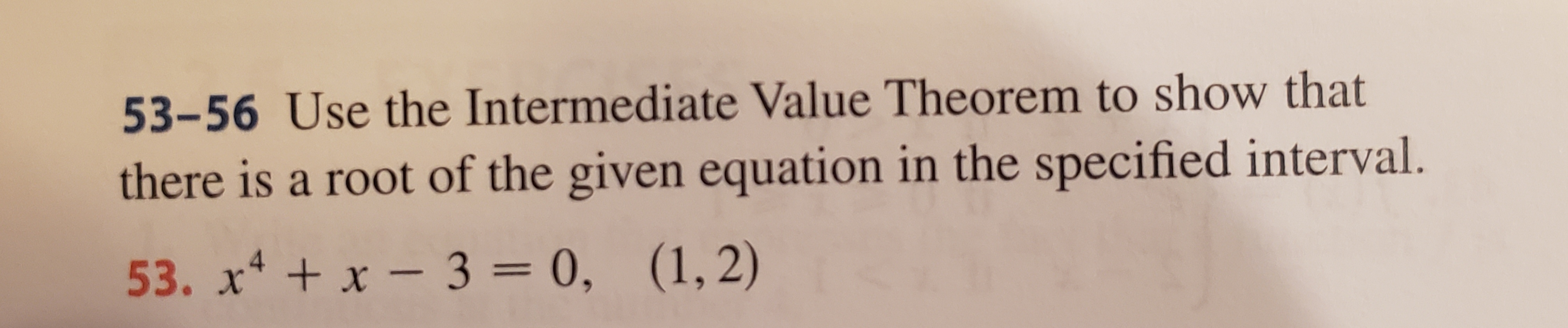# 53-56 Use the Intermediate Value Theorem to show thatthere is a root of the given equation in the specified interval.53. x4x-3 = 0, (1,2)

Question
12 viewshelp_outlineImage Transcriptionclose53-56 Use the Intermediate Value Theorem to show that there is a root of the given equation in the specified interval. 53. x4x-3 = 0, (1,2) fullscreen
check_circle

Step 1

We can prove that f(x) has a root between (a, b) if we can shown that f(a) and f(b) are different in sign.

Step 2

In this case, f(x) = x4 + x – 3 = 0;

(a, b) = (1, 2); Hence, a = 1; b = 2

Step 3

f(a) = f(1) = 14 + 1 – 3 = -1;

f(b) = f(2) = ...

### Want to see the full answer?

See Solution

#### Want to see this answer and more?

Solutions are written by subject experts who are available 24/7. Questions are typically answered within 1 hour.*

See Solution
*Response times may vary by subject and question.
Tagged in

### Functions